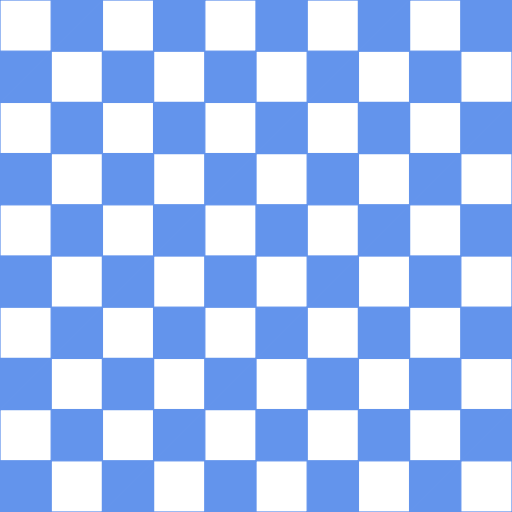# teaching machines

## Rotating Checkerboard

October 28, 2020 by . Filed under public, twoville.

Yesterday I posted a checkerboard that reorganized itself by translation. This one reorganizes by rotation.And here’s the Twoville source code.



step = 20

with export
size = [512, ~]

with time
start = 0
stop = step * 4
delay = 0.05

for r in -1..11
for c in -1..11
if abs(r) % 2 != abs(c) % 2
// Bottom right
with polygon()
stroke.color = :cornflower
stroke.size = 0.25
color = :cornflower
vertex().position = [c, r] * 10
vertex().position = [c + 1, r] * 10
vertex().position = [c + 1, r + 1] * 10
with rotate()
pivot = [c + 1, r] * 10
0 -> step * 1 -> t
degrees = 0
t -> step * 2 -> step * 3 -> t
degrees = -90
t -> step * 4 //-> step * 5 -> t
degrees = -180
// Top left
with polygon()
stroke.color = :cornflower
stroke.size = 0.25
color = :cornflower
vertex().position = [c, r] * 10
vertex().position = [c + 1, r + 1] * 10
vertex().position = [c, r + 1] * 10
with rotate()
pivot = [c, r + 1] * 10
0 -> step * 1 -> t
degrees = 0
t -> step * 2 -> step * 3 -> t
degrees = -90
t -> step * 4 //-> step * 5 -> t
degrees = -180

var twovilleDiv = jQuery('#twoville_rotate_checkers');
twovilleDiv.closest('pre').replaceWith(twovilleDiv);
document.getElementById('twoville_form_rotate_checkers').submit();

step = 20

with export
size = [512, ~]

with time
start = 0
stop = step * 4
delay = 0.05

for r in -1..11
for c in -1..11
if abs(r) % 2 != abs(c) % 2
// Bottom right
with polygon()
stroke.color = :cornflower
stroke.size = 0.25
color = :cornflower
vertex().position = [c, r] * 10
vertex().position = [c + 1, r] * 10
vertex().position = [c + 1, r + 1] * 10
with rotate()
pivot = [c + 1, r] * 10
0 -> step * 1 -> t
degrees = 0
t -> step * 2 -> step * 3 -> t
degrees = -90
t -> step * 4 //-> step * 5 -> t
degrees = -180
// Top left
with polygon()
stroke.color = :cornflower
stroke.size = 0.25
color = :cornflower
vertex().position = [c, r] * 10
vertex().position = [c + 1, r + 1] * 10
vertex().position = [c, r + 1] * 10
with rotate()
pivot = [c, r + 1] * 10
0 -> step * 1 -> t
degrees = 0
t -> step * 2 -> step * 3 -> t
degrees = -90
t -> step * 4 //-> step * 5 -> t
degrees = -180